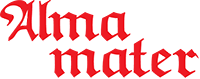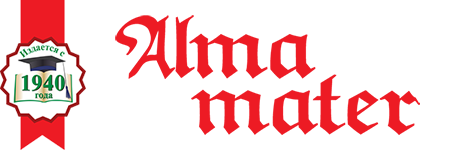ISSN 1026-955X
Vestnik Vysshey Shkoly (Higher School Herald)
The best way to learn all about Higher Education
Languages

=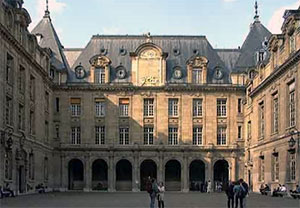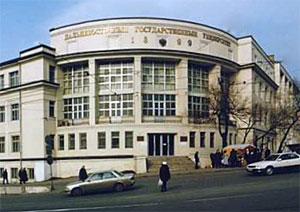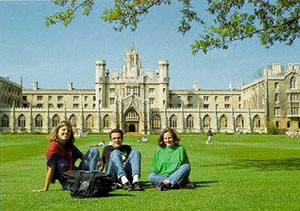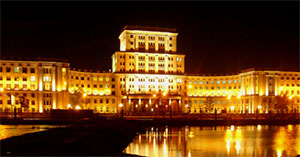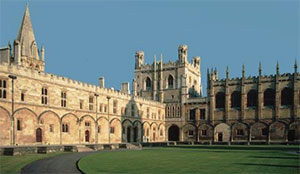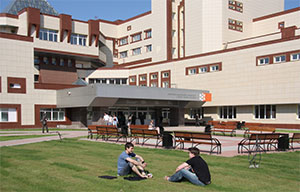# “Rhetorical shell” in Art rational reasoning of the Higher course mathematics for non-mathematical students

V.A. Erovenko
80,00 Р

UDC 378::51+808

Valery A. Erovenko, Dr. Sc. (Physics & Mathematics), Professor of the Department of General Mathematics and Computer Science at Belorussian State University, Minsk, e-mail: erovenko@bsu.by

The article discusses how non-mathematical students learn to understand mathematics, or rather help understand, in order to form their general mathematical culture of cognition. Thereby, their mathematical thinking becomes a rational and communicative phenomenon, so elements of higher mathematics should become one of the main courses in the fundamental training of future specialists. With his rhetoric and understood argumentation, a creative teacher leads the student to correct reasoning. The understanding of higher mathematics is characterized by the ability to establish logical connections between accumulated and new knowledge. The cognitive ability to prove is very much demanded by the course of mathematics in geographical and environmental research.

Key words: rhetoric in a mathematical lecture, rational reasoning, higher mathematics for students-geographers.

References

1. Karpovich, V.N. Formal logic, rhetoric and rational reasoning. Siberian Philosophical Journal. 2019. Vol. 17. No. 1. P. 5–16.

2. Erovenko, V.A. The philosophy education of Cicero’s and the place of rhetoric in the teaching of mathematics. Liberal Arts in Russia. 2017. Vol. 6. No. 2. P. 109–119.

3. Rubtsov, V.A., Stepin, A.G. Mathematics and geography (a modern view of the penetration of mathematics into geographical knowledge). Bulletin of the Association of Russian Social Geographers. 2016. No. 5. P. 255–269.

4. Erovenko, V.A. University rhetoric as a cognitive means of liberating mathematical thinking. Teacher of the XXI century. 2020. No. 1–1. P. 9–18.

5. Michailova, N.V. Rational essence of mathematical education and the problem of substantiation. Mathematical structures and modeling. 2019. No. 1. P. 38–46.